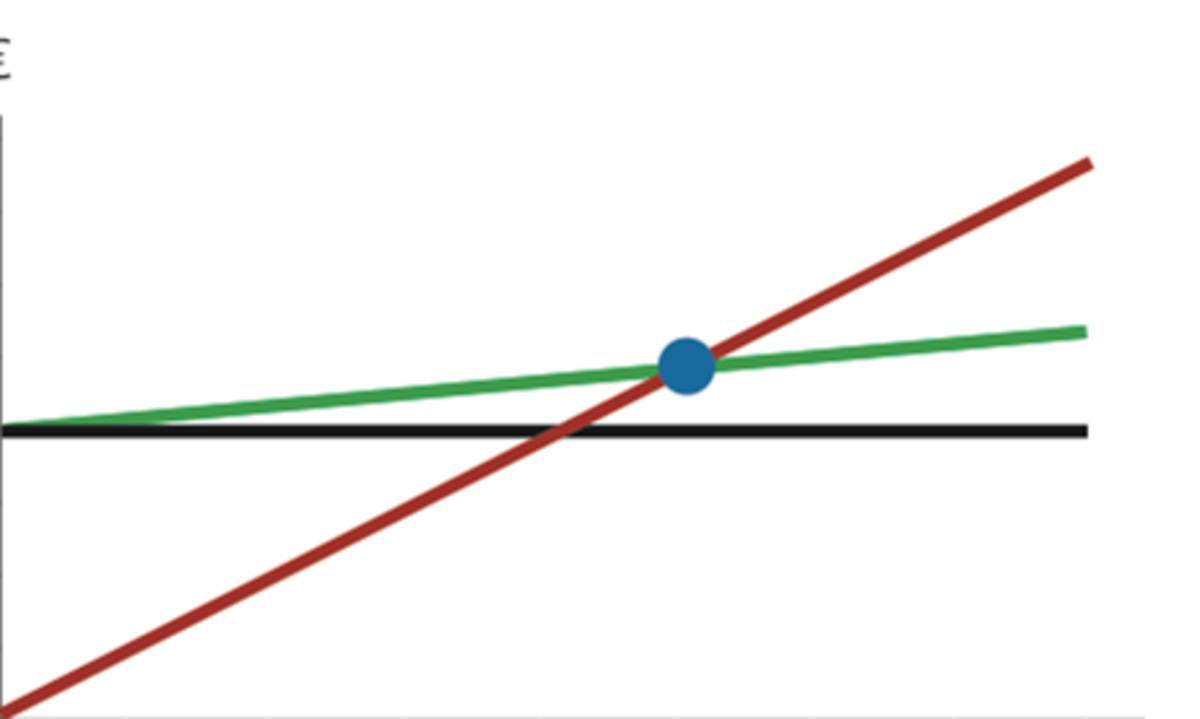Updated date:

# How to Complete a Break-Even Analysis in Production: Between Processes

Joshua has work experience in aerospace/aluminum manufacturing & distribution. He received his BBA in accounting from Kent State University.Finding the break-even point of a process can aid in understanding constraints, costs, and production planning. Created by Joshua Crowder

## What Is Break-Even Analysis?

A break-even analysis can calculate the point at which revenues equal expenses, among a myriad of other applications. Here, I discuss breakeven analysis specifically for process analysis. This application is used to compare processes in a production setting.

With break-even analysis, the costs of different processes can be compared to figure out what quantities need to be produced to make that process worth putting in place. The costs involved in a process must first be separated into variable and fixed costs due to their nature.

Fixed costs do not change with the quantity of output and do not zero when production is zero. For example, if we stop producing, the fixed cost remains. Fixed cost examples include rent, insurance, and loan payments.

In the example used in this text, fixed cost will be the cost of equipment. Variable costs change with the increase or decrease of output. Variable costs can be labor rates, shipping costs, and direct materials costs.

For simplicity, variable cost will be considered the labor cost per hour only and will be transferred into a cost per unit of product.

## Break-Even Analysis Example

This example examines different processes within a pastry company. Suppose that there are different ways to produce pastries. The three ways that pastries could be produced are outlined below.

### Process 1: Manual Cutting

One process (process 1) involves a worker cutting pastries by hand with a tool. The work performed does not require the assistance of a machine. When using this process, a worker can cut out 120 pastries per hour while being paid a rate of \$12 per hour.

### Process 2: Automated Machine

Another process (process 2) used is a machine called the Demag 2000. This machine has a fixed cost of \$10,000 and can cut out 600 pastries per hour.

### Process 3: Automated Machine

The third process involves another machine called the Demag 3000. This equipment has a fixed cost of \$15,000 and can cut out 900 pastries per hour.

If we know that the bakery always meets its target of 200,000 pastries per year, we can find out how long it will take to recoup the costs associated with using a machine rather than just using labor. The labor cost per pastry equals the pastry cutter's hourly wage rate divided by the output of that process.

## Step 1

The first thing we want to do is find where process 1 and process 2 intersect. To do so, each variable cost must be multiplied by Q.

Additionally, fixed costs need to be added to process 2. Keep in mind that process 1 has no fixed cost since there is no capital investment involved.

Next, each process calculation needs to set to equal each other. The equation is shown below:

p1 = p2

\$.1 x Q = \$10,000 + \$.02 x Q

\$.1Q - \$.02Q = \$10,000

\$.08Q = \$10,000

Q =125,000

This break-even point (BEP) shows the point at which both processes will be creating the same output at the same cost. So, if the company produces quantity a of 200,000 per year like they plan, then it will take the company 7.5 months 125,000/ 200,000 to recover the costs of purchasing a new machine (process 2). When comparing processes 1 and 2, it is better to use process 1 when under 125,000 pastries need to be produced and process 2 if more than 125,000 pastries need to be produced.

## Step 2

Next, we can solve the breakeven point for process 2.

p2 = p3

\$10,000 + \$.02 X Q = \$15,000 + \$.0133 x Q

\$10,000 + \$.02Q = \$15,000 + \$.0133333Q

\$.006667Q = \$15,000

\$.006667Q = \$5,000

When comparing the two automated processes, we see that process is more useful if 750,000 pastries are produced.

## How to Create a Break-Even Graph

When completing a break-even analysis, it's always nice to have a chart to help you reinforce your findings to an audience. A chart can be made easily in Microsoft Excel.

While in excel, make four columns—one for your X-Axis (Quantity) and three for your Y-Axes (Cost).

For the quantity column, start at 0 and add 100,000, 200,000, 300,000 . . . until you reach 1 million.

Now, for p1, plug 0 into the equation \$.10 X Q you will get zero.

For p2 when you plug in 0, you get 10,000 (\$10,000 +\$.02 x Q).

When plugging in for p3, you get 15,000 (\$15,000 + \$.0066 x Q).

Continue to calculate for p1, p2, and p3 while plug in each 100,000 for Q.

(hint: in excel if you complete a few rows you can highlight those rows and use the fill handle to complete the table). You should have a table like the one shown below.

## Creating The Graph

To create the graph, you must select all the data in the table click on the insert tab, then click on the scattered line selection that I circled in the photo. After creating the graph, I suggest adding a title and reducing the axis maximum quantity to show a better representation of the breakeven points. If you would like a copy of the table or graph you can download a link to the spreadsheet here.